# 【排序算法】图解桶排序

## 前言## 桶排序思想

• 桶：若干个桶，说明此类排序将数据放入若干个桶中。
• 桶：每个桶有容量，桶是有一定容积的容器，所以每个桶中可能有多个元素。
• 桶：从整体来看，整个排序更希望桶能够更匀称，即既不溢出(太多)又不太少。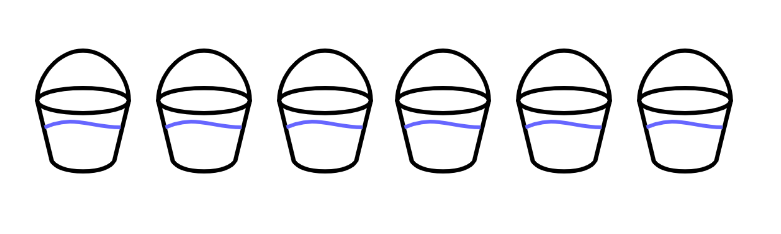• 将待排序的序列分到若干个桶中，每个桶内的元素再进行个别排序。
• 时间复杂度最好可能是线性O(n)，桶排序不是基于比较的排序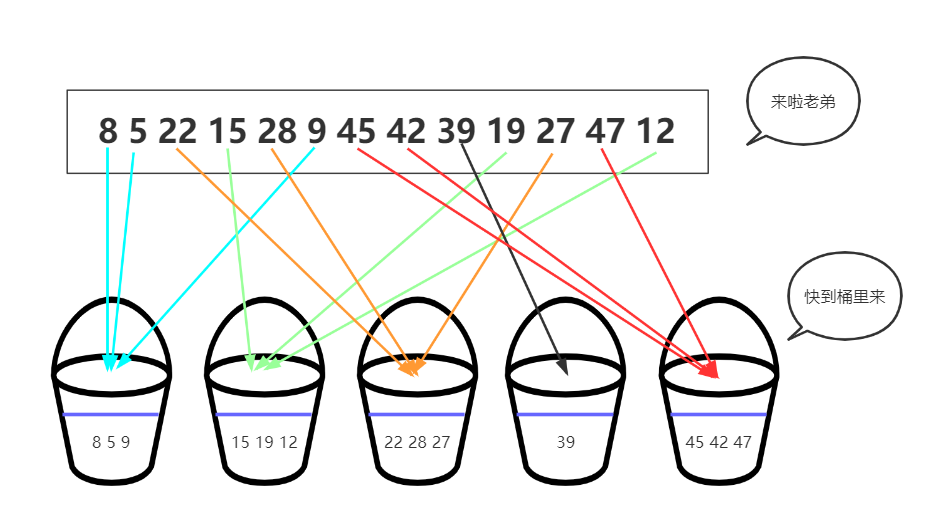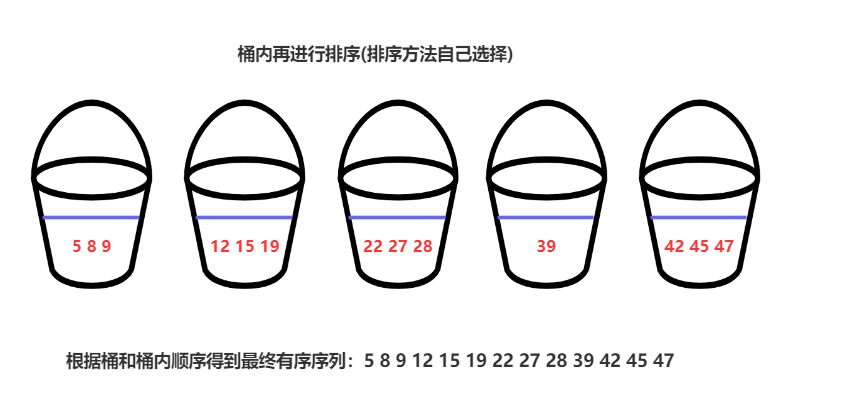## 桶排序算法分析### 时间复杂度分析

• 1.遍历处理每个元素，O(n)级别的普通遍历
• 2.每个桶内再次排序的时间复杂度总和

• 如果桶内元素分配较为均匀假设每个桶内部使用的排序算法为快速排序，那么每个桶内的时间复杂度为(n/m) log(n/m)。有m个桶，那么时间复杂度为m * (n/m)log(n/m)=n (log n-log m).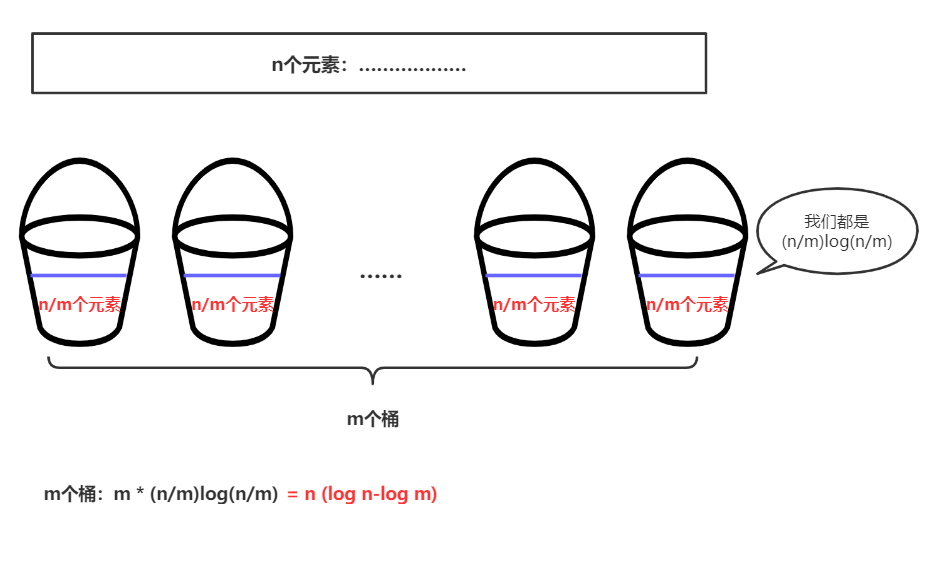所以最终桶排序的时间复杂度为：O(n)+O(n*(log n- log m))=O(n+n*(log n -log m)) 其中m为桶的个数。我们有时也会写成O(n+c),其中c=n*(log n -log m);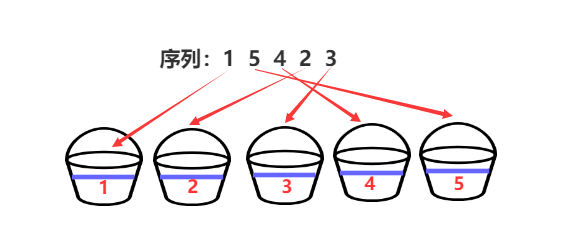### 桶排序适用情况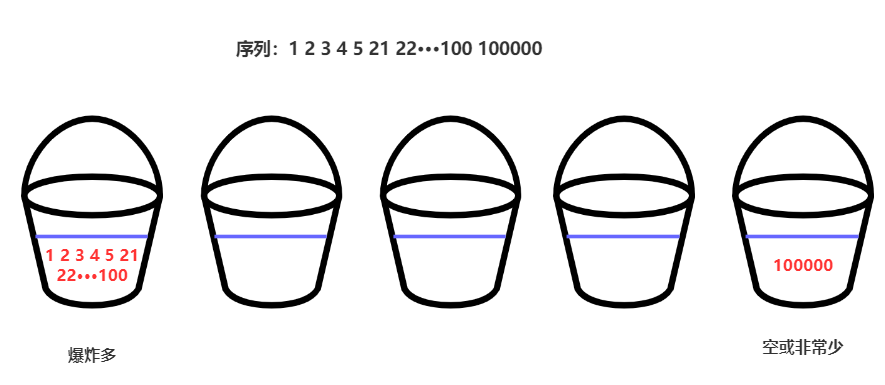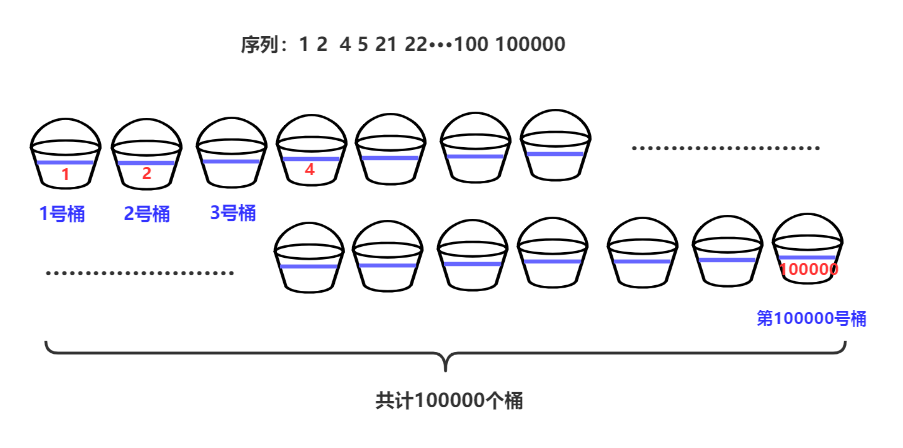## 实现一个桶排序

import java.util.ArrayList;
import java.util.List;
//微信公众号：bigsai
public class test3 {
public static void main(String[] args) {
int a[]= {1,8,7,44,42,46,38,34,33,17,15,16,27,28,24};
List[] buckets=new ArrayList;
for(int i=0;i<buckets.length;i++)//初始化
{
buckets[i]=new ArrayList<Integer>();
}
for(int i=0;i<a.length;i++)//将待排序序列放入对应桶中
{
int index=a[i]/10;//对应的桶号
}
for(int i=0;i<buckets.length;i++)//每个桶内进行排序(使用系统自带快排)
{
buckets[i].sort(null);
for(int j=0;j<buckets[i].size();j++)//顺便打印输出
{
System.out.print(buckets[i].get(j)+" ");
}
}
}
}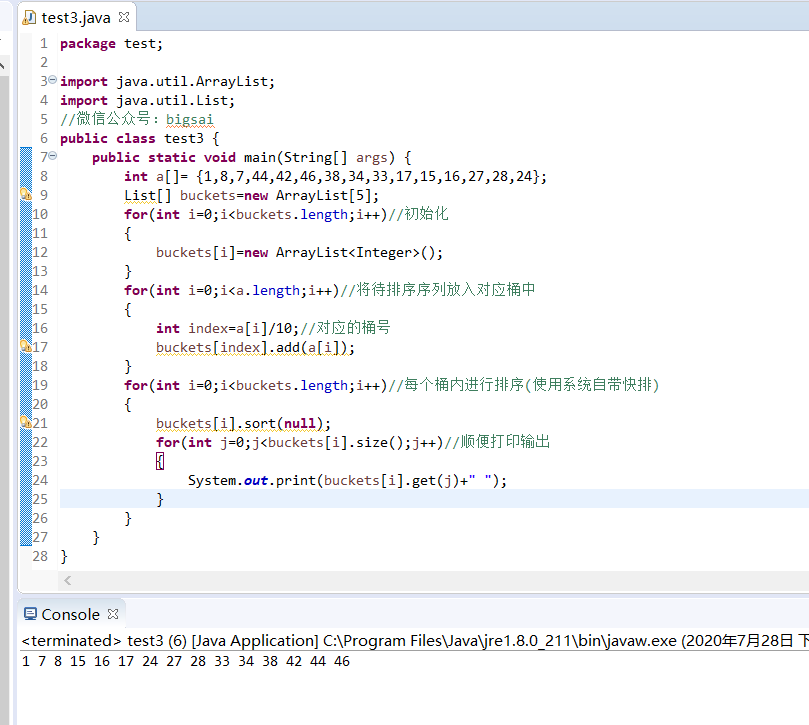## 结语Big sai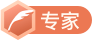CSDN认证博客专家 数据结构与算法 爬虫 Java

12-01
10-2139304-05165
02-1221
04-01607
02-23813
07-12109
07-19
10-292225
10-3122
05-024792点击重新获取扫码支付余额充值